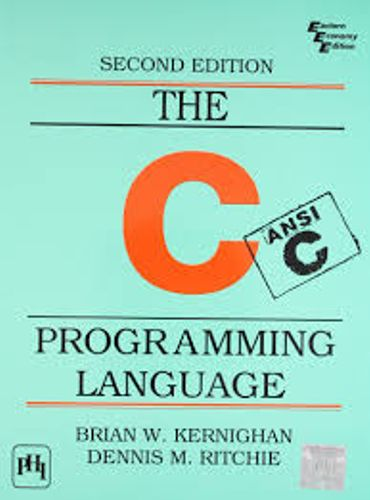# Conditions

### Decision Making

In life, we all have to make decisions. In order to make a decision we weigh out our options and so do our programs.

Here is the general form of the decision making structures found in C Programming Language.

``````int target = 10;
if (target == 10) {
printf("Target is equal to 10");
}
``````

### The `if` statement

The `if` statement allows us to check if an expression is `true` or `false`, and execute different code according to the result.

To evaluate whether two variables are equal, the `==` operator is used, just like in the first example.

Inequality operators can also be used to evaluate expressions. For example:

``````int foo = 1;
int bar = 2;

if (foo < bar) {
printf("foo is smaller than bar.");
}

if (foo > bar) {
printf("foo is greater than bar.");
}
``````

We can use the `else` keyword to exectue code when our expression evaluates to `false`.

``````int foo = 1;
int bar = 2;

if (foo < bar) {
printf("foo is smaller than bar.");
} else {
printf("foo is greater than bar.");
}
``````

Sometimes we will have more than two outcomes to choose from. In these cases, we can “chain” multiple `if` `else` statements together.

``````int foo = 1;
int bar = 2;

if (foo < bar) {
printf("foo is smaller than bar.");
} else if (foo == bar) {
printf("foo is equal to bar.");
} else {
printf("foo is greater than bar.");
}
``````

You can also nest `if` `else` statements if you like.

``````int peanuts_eaten = 22;
int peanuts_in_jar = 100;
int max_peanut_limit = 50;

if (peanuts_in_jar > 80) {
if (peanuts_eaten < max_peanut_limit) {
printf("Take as many peanuts as you want!\n");
}
} else {
if (peanuts_eaten > peanuts_in_jar) {
printf("You can't have anymore peanuts!\n");
}
else {
printf("Alright, just one more peanut.\n");
}
}
``````

Two or more expressions can be evaluated together using logical operators to check if two expressions evaluate to `true` together, or at least one of them. To check if two expressions both evaluate to `true`, use the AND operator `&&`. To check if at least one of the expressions evaluate to `true`, use the OR operator `||`.

``````int foo = 1;
int bar = 2;
int moo = 3;

if (foo < bar && moo > bar) {
printf("foo is smaller than bar AND moo is larger than bar.");
}

if (foo < bar || moo > bar) {
printf("foo is smaller than bar OR moo is larger than bar.");
}
``````

The NOT operator `!` can also be used likewise:

``````int target = 9;
if (target != 10) {
printf("Target is not equal to 10");
}
``````

## Home Work

In this exercise, you must construct an `if` statement inside the `guessNumber` function statement that checks if the number `guess` is equal to 555. If that is the case, the function must print out using `printf` “Correct. You guessed it!”. If `guess` is less than 555, the function must print out using `printf` “Your guess is too low.” If `guess` is greater than 555, the function must print out using `printf` “Your guess is too high.”

Click on image to Download C Programming Language Book :